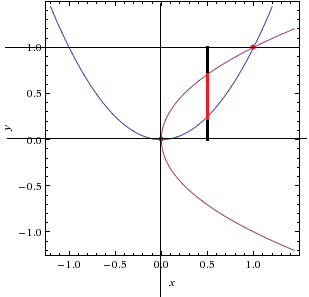# Solids of revolution volume

## Homework Statement

Volume of the region bounded by y = x^2 and x = y^2 about y = 1

## Homework Equations

$\pi r^2$

## The Attempt at a Solution

So the functions look something like this:I decided to use method of washers with respect to x.

The radius if the center is at y = 1 of the washers is going to be $\sqrt{x} - x^2$ and the inside space is going to have a radius of $1 - \sqrt{x}$. So we want to subtract these 2 volumes:

$\pi\int_{0}^{1}(\sqrt{x} - x^2)^2 dx - \pi\int_{0}^{1}(1 - \sqrt{x})^2 dx$

So I get:

$\pi\int_{0}^{1}(\sqrt{x} - x^2)^2 dx - \pi\int_{0}^{1}(1 - \sqrt{x})^2 dx \\ \pi\int_{0}^{1}(x - 2x^{\frac{5}{2}} + x^4) - (1 - 2x^\frac{1}{2} + x) dx \\ \pi\int_{0}^{1}(-2x^{\frac{5}{2}} + x^4 - 1 + 2x^\frac{1}{2}) dx \\ \pi[(\frac{-4}{7}x^{\frac{7}{2}} + \frac{x^5}{5} - x + \frac{4}{3}x^\frac{3}{2})]_{0}^{1} \\ \pi(-\frac{4}{7} + \frac{1}{5} - 1 + \frac{4}{3}) \\ \pi\frac{-4}{105}$

Not sure how I get a negative number. What did I do wrong?

## Answers and Replies

eumyang
Homework Helper
I decided to use method of washers with respect to x.

The radius if the center is at y = 1 of the washers is going to be √x - x2 and the inside space is going to have a radius of $1 - \sqrt{x}$.
I don't think the outer radius is correct. We want the distance from the curve y = x2 to the line y = 1. Shouldn't it be 1 - x2?

Oh, I was using the wrong radius...thanks, I see it now